# IBPS PO Prelims Reasoning Question Paper 2019

• Last Updated : 25 Mar, 2022

#### Direction (1-5): Study the following information carefully and answer the questions given below:

Eight persons M, N, O, P, Q, R, S, and T are sitting around a rectangular table in such a way that four persons sit on each of the four corners of the table and the other four persons sit on the middle of each side. The one who sits at the corner of the table faces outside the center of the table and the one who sits at the middle side of the table faces towards the center of the table. Persons sitting on opposite sides are sitting opposite to each other. The person who sits second to the left of R sits second to the right of the one who sits to the immediate right of Q. Only one person sits between R and O. Q does not sit near to O. Either M sits second to the left of T or second to the right of T. One person sits between M and O. N sits fourth to the left of S. P sits on one of the corners. S is not an immediate neighbor of R.

Solution (1-5):Q1. Four of the following five are alike in a certain way and hence they form a group. Who among the following does not belong to that group?

(a) Q

(b) S

(c) P

(d) T

(e) N

Solution: d

T does not belong to that group.

Q2. Who among the following sits third to the right of P?

(a) Q

(b) T

(c) O

(d) M

(e) S

Solution: b

T is third to the right of P.

Q3. What is the position of R with respect to S?

(a) Third to the right

(b) Second to the right

(c) Second to the left

(d) Third to the left

(e) None of these

Solution: a

R is third to the right of S.

Q4. How many persons sit between P and R when counted to the right of P?

(a) One

(b) None

(c) Two

(d) Three

(e) Four

Solution: b

None of the people sits between P and R.

Q5. Who among the following faces to O?

(a) T

(b) N

(c) S

(d) Q

(e) None of these

Solution: a

T is facing O.

Q6. In the word ‘INVITED’, how many pairs of the letters have the same number of letters between them in the word as in the alphabet?

(a) One

(b) Two

(c) Three

(d) Four

(e) More than four Direction

Solution: bTwo Pairs of letters have the same number of letters between them in the word.

#### Direction (7-9): Study the following information carefully and answer the questions given below:

Seven members are living in the family. O is the daughter of U. M is the brother of T. S is the mother-in-law of A. M is married to A. M is the uncle of O. N is the father of M and he has only two children.

#### Solution (7-9):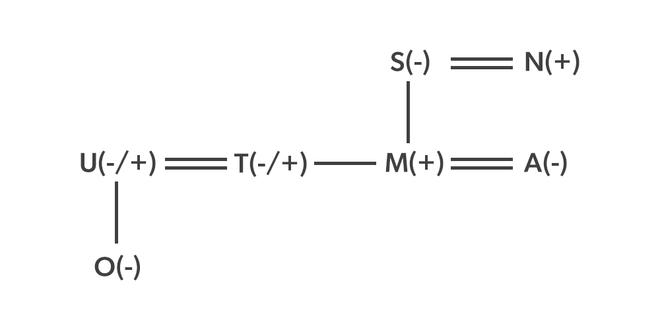Q7. What is the relation of M with respect to U?

(a) Brother

(b) Sister in law

(c) Mother

(d) Brother in law

(e) Aunt

Solution: d

M is a Brother in law of U.

Q8. If C is the brother of M, then what is the relation of C with respect to O?

(a) Aunt

(b) Uncle

(c) Father

(d) Mother

(e) Sister

Solution: b

C is the uncle of O.

Q9. If U is the father of O, then what is the relation of T with respect to U?

(a) Mother

(b) Husband

(c) Father

(d) Father in law

(e) Wife

Solution: e

T is a wife of U.

Q10. If “3985436247’’ 1 is added to all the even numbers and 1 is subtracted from all the odd numbers, then what is the sum of the digits which is sixth from the left end and fourth from the right end?

(a) 9

(b) 12

(c) 11

(d) 10

(e) None of these

Solution: a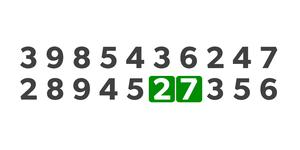The sum of digits is 9.

#### Direction (11-15): Study the following information carefully and answer the questions given below:

Ten persons A, B, C, D, E, F, G, H, I, and J were born on two different dates 13 and 26 of five different months viz. March, May, June, September, and November but not necessarily in the same order. A was born on the 13th of the month which has 31 days. Three persons were born between A and E. One person was born between E and G. Four persons were born between E and D. The number of persons was born after D is the same as the number of persons born before C. B was born before I but not in the month of March. I was born before E. J was born before H on the same date but in different months.

#### Solution (11-15):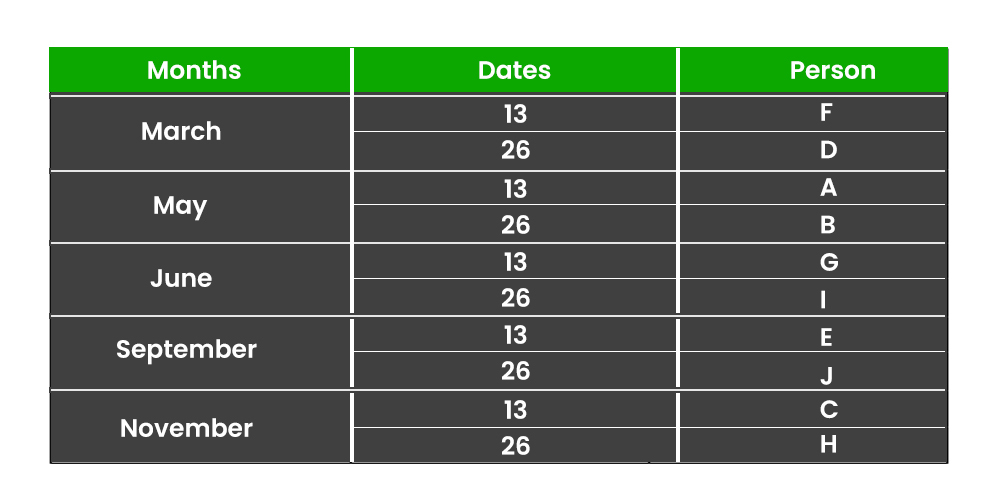Q11. How many persons were born after J?

(a) None

(b) Two

(c) More than five

(d) Four

(e) Five

Solution: b

Two persons born after J.

Q12. Four of the following five are alike in a certain way and hence they form a group. Which one of the following does not belong to that group?

(a) F-A

(b) G-E

(c) H-A

(d) E-C

(e) B-D

Solution: c

H-A does not belong to that group.

Q13. How many persons were born between I and C?

(a) One

(b) Three

(c) Four

(d) Two

(e) None of these

Solution: d

Two persons were born between I and C.

Q14. The number of persons was born after E is the same as the number of persons born before ___.

(a) B

(b) G

(c) F

(d) J

(e) None of these

Solution: a

The number of persons was born after E is the same as the number of persons born before B.

Q15. Which of the following is the odd one?

(a) F

(b) A

(c) G

(d) E

(e) H

Solution: e

H is different among them.

#### Q16. Statements: S>M>R=F; C<V=B>N; F ≥Y<X ≤ C

Conclusion:

I: S>X

II: Y<B

Solution: b

Conclusion:

I: S>X(False)

II: Y<B(True)

Hence, only conclusion II follows.

#### Q17. Statements: L>C<G<H≤J, T<X<C≤V;

Conclusion:

I: T<G

II: X<J

Solution: e

Conclusion:

I: T<G(True)

II: X<J(True)

Hence, both conclusions, I and II follow.

#### Q18. Statements: O≥W≥E; V<Y≤U≤I; E=S<V;

Conclusion:

I: O≥V

II: S≤I

Solution: d

Conclusion

I: O≥V (False)

II: S≤I (False)

Hence, neither conclusion I nor II follows.

#### Q19. Statements: H>D<Z<X<E, B<S<D=F>G;

Conclusion

I: B<E

II: E≥G

Solution: a

Conclusion

I: B<E(True)

II: E≥G(False)

Hence, the only conclusion I follows.

#### Q20. Statements: C=V>B>K; N>B<S=D<F<H; G ≥ Y ≥ Q= V

Conclusion

I: G >K

II: K<H

Solution: e

Conclusion

I: G>K (True)

II: K<H (True)

Hence, both conclusions, I and II follow.

#### In a certain code language:

• “not talk between only” is coded as “lg pf ro mi”
• “only should be helpful” is coded as “ro tc ac jq”
• “not be time standard” is coded as “mi dg tc pa”
• “Focus between standard hours” is coded as “hy dg pf ks”

#### Solution (21-25):

Q21. What is the code for “not time” in the given code language?

(a) pf ro

(b) ro mi

(c) mi pa

(d) jq pf

(e) None of these

Solution: c

‘mi pa’ is the code of “not time”.

Q22. What is the code for “standard” in the given code language?

(a) dg

(b) ac

(c) jq

(d) mi

(e) None of these

Solution: a

‘dg’ is the code of “standard”.

Q23. What is the code for “only” in the given code language?

(a) lg

(b) ro

(c) tc

(d) pa

(e) None of these

Solution: b

The code for “only” is ‘ro’.

Q24. The code ‘tc’ is coded as which of the following word?

(a) not

(b) time

(c) focus

(d) be

(e) None of these

Solution: d

The word ‘be’ is coded as ‘tc’.

Q25. What may be the possible code for “focus real” in the given code language?

(a) ks ze

(b) ro hy

(c) hy tr

(d) Either (a) or (c)

(e) pf me

Solution: d

Either options (a) or (c) may be the possible code for “focus real” in the given code language.

#### Direction (26-29): Study the following information carefully and answer the questions given below:

Six persons are sitting in a row facing to the north and they have different ages but not necessarily in the same order. T sits third from the one who sits on one of the ends of the row. The one who is 12 years old sits second to the right of T.  The number of persons sitting to the right of the one who is 12 years old is the same as the number of persons sitting to the left of V. W sits second to the right of the one who is 6 years old. Two persons sit between V and X, whose age is twice that V. U sits to the right of S. The one who is 36 years old sits third to the left of the one who is 15 years old. The age of W is 18 years.

#### Solution (26-29):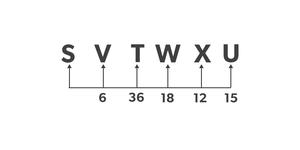Q26. If S is 3 years older than T, then which of the following is the age of S?

(a) 9 Years

(b) 33 Years

(c) 21 Years

(d)39 Years

(e) None of these

Solution: d

Age of S is 39 years

Q27. What is the total age of T and X?

(a) 44

(b) 48

(c) 18

(d) 42

(e) 27

Solution: b

The total age of T and X is 48.

Q28. How many persons sit between S and V?

(a) More than three

(b) Three

(c) Two

(d) One

(e) None

Solution: e

None persons sit between S and V.

Q29. Who among the following sits at the extreme end of the row?

(a) T

(b) X

(c) U

(d) W

(e) V

Solution: c

U sits end of the row.

#### Direction (30-34): Study the following information carefully and answer the questions given below:

Seven persons were born in different years i.e. 1953, 1963, 1968, 1976, 1990, 1993, 1998. 2019 is the base year for measuring the age of all persons. No more than two persons were born before H. One person was born between L and O and none of them have even-numbered age. The number of people who were born before M is equal to the number of people who were born after Y. Difference between the age of N and O is not less than 20 years. N was born before Y and after G. Either H or M have odd-numbered age.

#### Solution (30 -34):

Q30. In which of the following year N was born?

(a) 1953

(b) 1968

(c) 1990

(d) 1998

(e) 1976

Solution: e

N was born in 1976.

Q31. What is the sum of the age of G and O?

(a) 92

(b) 69

(c) 87

(d) 50

(e) None of these

Solution: c

The sum of the age of G and O is 87 years.

Q32. Who among the following is the oldest person?

(a) H

(b) Y

(c) G

(d) N

(e) None of these

Solution: c

G is the oldest person among them.

Q33. Which of the following pair of combinations is true?

(a) H- 29

(b) Y-26

(c) G-56

(d) O-43

(e) None is true

Solution: b

Y – 26 pair of combination is true.

Q34. How many persons are younger than M?

(a) None

(b) One

(c) Two

(d) Three

(e) More than three

Solution: e

More than three-person younger than M.

Q35. In the given word ‘WANTED’, in which consonant changed into its previous letter and the vowel into its next letter (according to the alphabetical order) and then all letters are arranged in alphabetical order from left to right then which of the following letter is fourth from the left end?

(a) F

(b) V

(c) B

(d) M

(e) S

Solution: d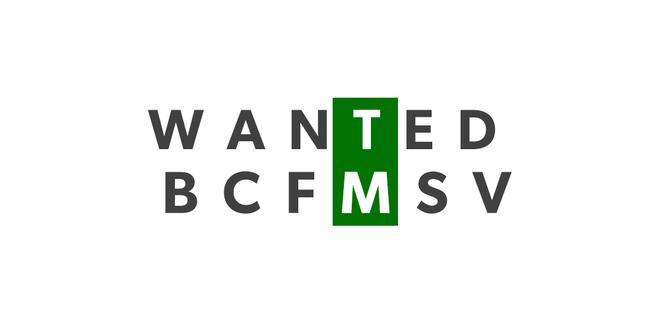M is the fourth from the left end.

My Personal Notes arrow_drop_up
Recommended Articles
Page :